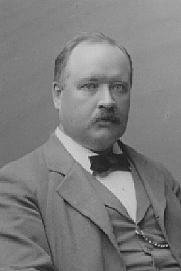Q10 - the facts Reaction rates do not always double for 10oC temperature rise. Home | Chemistry

Q10 – rubber-stamp ‘thinking’.

‘Reaction rates double for every 10oC rise in temperature’ – I expect that you’ve heard this statement. It is, for some reason, very popular with those studying biology; it is there that you find Q10, the ratio of reaction rate at temperature T to that at T + 10. The doubling is usually offered as a fact of chemical (or biochemical) life; such a pity, then, that it isn’t true.

As originally promulgated in university texts it was ‘Reaction rates roughly double or triple…’; but in the (ever-increasing) sanitising found in A level texts a number of fairly important words were jettisoned. Like ‘roughly’, ‘double or triple’. (Yes, guilty; if you’ve read my Philip Allan texts there are numerous omissions there, and no, I don’t like that.) A number of other important caveats disappeared too, so let’s examine the ideas further.

The Arrhenius equation.

The rate of a chemical reaction is described by an empirically-determined equation of the form

rate = k [A]x [B]y

where k is the rate constant, [A] and [B] are the molar concentrations of the species A and B, and x and y are the orders of reaction with respect to A and to B. There may, of course, be only A, or there may be more than two species involved.

The concentrations do not depend significantly upon temperature; the term that changes with a change in temperature  is k. That is why all experiments on reaction rates must be done at constant temperature. The temperature-dependence of k is described by the Arrhenius equation (S Arrhenius, 1889,  building on earlier work of J H van’t Hoff (1884)):

ln (k2/k1) = (Ea/R) (1/T1 – 1/T2)

where k1 and k2 are the rate constants at (absolute, i.e. Kelvin) temperatures T1 and T2, Ea is the activation energy for the reaction in J mol-1, and R is the gas constant, 8.314 J K-1 mol-1.

The term that is Q10 is k2/k1, and this is how I shall refer to it from now on.

Two important points

What is not usually stated in A level kinetics:

• k2/k1 depends on the value of Ea;
• k2/k1 depends on temperature – Q10 is not constant. Increasing the temperature decreases k2/k1 .

A few calculations.

A value for Ea:
Firstly we shall find the value of Ea that would give a doubling of reaction rate between 0oC and 10oC, i.e. for k2/k1 = 2. Then with this value of Ea we shall see what the effect is on k2/k1 at two different 10oC ranges. Remember that the temperatures in the Arrhenius equation must be in K.

For the calculation of Ea we take k2/k1 = 2, T1 = 273 K, T2 = 283 K, R = 8.314 J K-1 mol-1:

 Arrhenius: ln (k2/k1) = (Ea/R) (1/T1 – 1/T2) Therefore: ln (k2/k1) / (1/T1 – 1/T2)  = Ea/R Substituting the values given: (8.314 x ln 2) / (1/273 – 1/283)  = Ea thus (8.314 x 0.693) / (1/273 – 1/283) = Ea

and it is only arithmetic to show that Ea = 44,500 J mol-1 = 44.5 kJ mol-1.

The effect of increasing the temperature on k2/k1:
If we now use this value of Ea and find the value of k2/k1 at different temperatures for this hypothetical reaction, i.e. evaluate

ln (k2/k1) = (44,500/8.314) (1/T1 – 1/T2)

more arithmetic shows the following (try it):

 T1/K T2/K k2/k1 273 283 2.00 373 383 1.45 473 483 1.26

Indeed the value of k2/k1, our old – but now unreliable – friend, Q10, falls as the temperature increases.

Would you need this in an A level exam? No. But at least you won’t fall into rubber-stamp thinking on this topic again.

Lastly – the rate of a reaction seems to be determined by Ea. This is true to a good approximation (unlike Q10); but in fact it is the free energy of activation that is the important factor. But that’s for another day. Ea is pretty good until then.Svante August Arrhenius
(1859 - 1927)